# k kiloh hectoda dekad decic centim milli 1000

• Slides: 8
Download presentation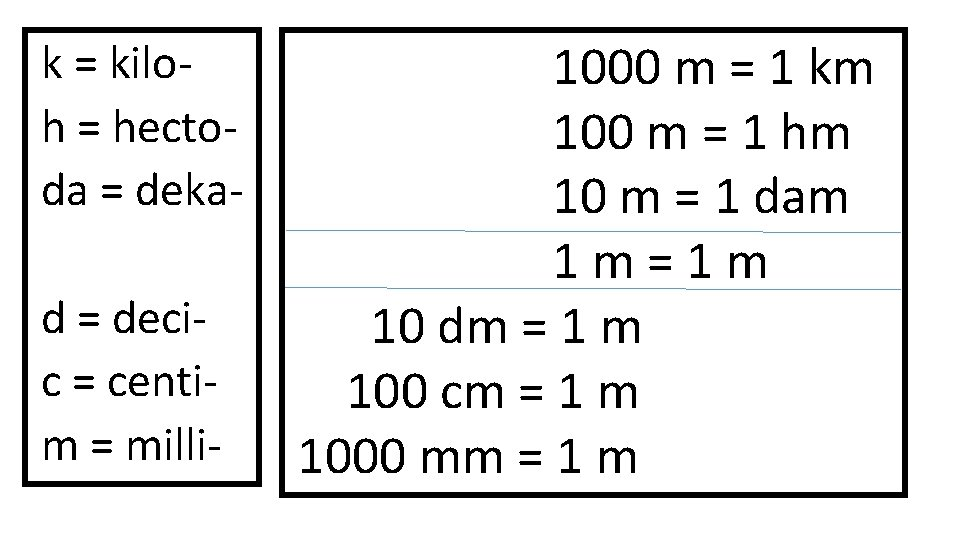k = kiloh = hectoda = dekad = decic = centim = milli- 1000 m = 1 km 100 m = 1 hm 10 m = 1 dam 1 m=1 m 10 dm = 1 m 100 cm = 1 m 1000 mm = 1 m1. Write down the number that will be converted. 2. Set up a conversion factor. 3. Put the same unit as the number being converted on the denominator of the conversion factor. 4. Write the base unit (meters, liters, grams, etc. ) 5. Place a “ 1” in front of the larger unit. 6. Determine how many of the smaller units can go into the larger unit. Place this number in front of the smaller unit. 7. Repeat steps 2 through 6 until the unit on the numerator matches the unit as the unit that you are solving for. 8. Multiply the equation out. You may find it easier to multiple all numerators together and all denominators together to create a fraction. Follow this by dividing the numerator by the denominator.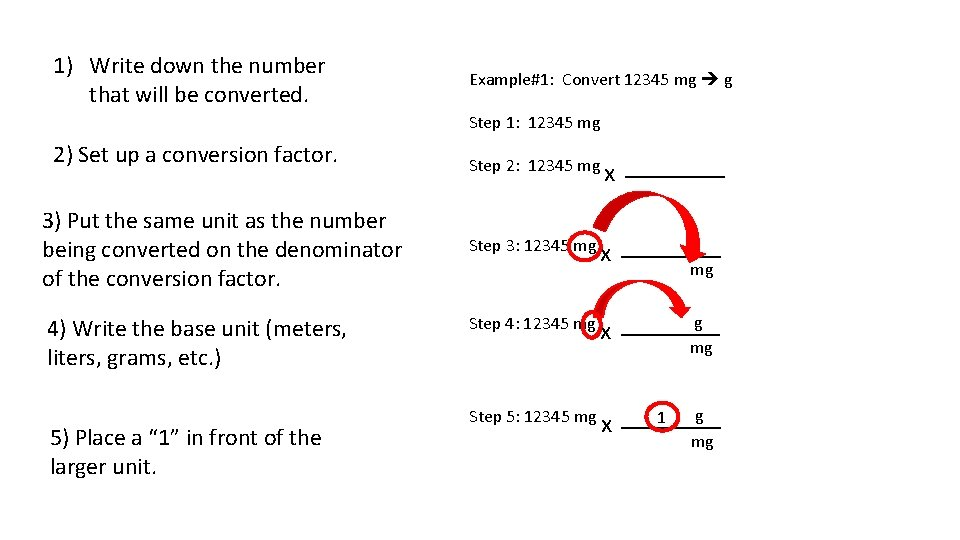1) Write down the number that will be converted. Example#1: Convert 12345 mg g Step 1: 12345 mg 2) Set up a conversion factor. 3) Put the same unit as the number being converted on the denominator of the conversion factor. 4) Write the base unit (meters, liters, grams, etc. ) 5) Place a “ 1” in front of the larger unit. Step 2: 12345 mg x _____ Step 3: 12345 mg x _____ x g _____ 1 Step 4: 12345 mg Step 5: 12345 mg mg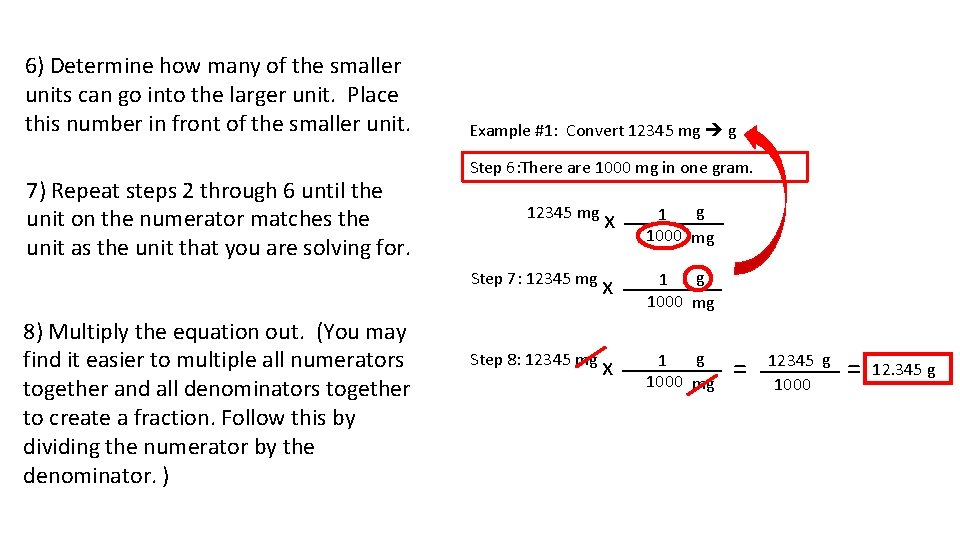6) Determine how many of the smaller units can go into the larger unit. Place this number in front of the smaller unit. 7) Repeat steps 2 through 6 until the unit on the numerator matches the unit as the unit that you are solving for. Example #1: Convert 12345 mg g Step 6: There are 1000 mg in one gram. 12345 mg Step 7: 12345 mg 8) Multiply the equation out. (You may find it easier to multiple all numerators together and all denominators together to create a fraction. Follow this by dividing the numerator by the denominator. ) Step 8: 12345 mg x g _____ 1 12345 g = ____ = 12. 345 g 1000 mg 1000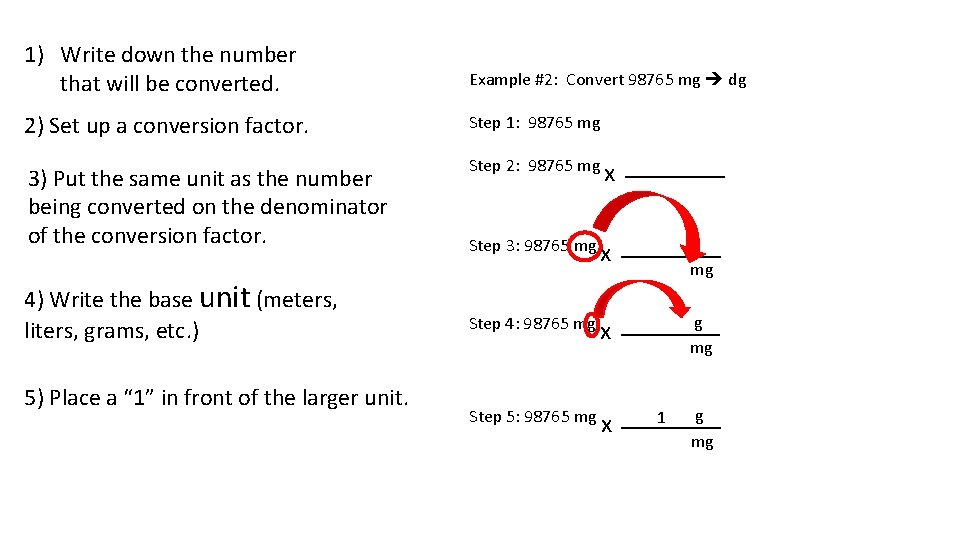1) Write down the number that will be converted. Example #2: Convert 98765 mg dg 2) Set up a conversion factor. Step 1: 98765 mg 3) Put the same unit as the number being converted on the denominator of the conversion factor. 4) Write the base unit (meters, liters, grams, etc. ) 5) Place a “ 1” in front of the larger unit. Step 2: 98765 mg x _____ Step 3: 98765 mg x _____ x g _____ 1 Step 4: 98765 mg Step 5: 98765 mg mg6) Determine how many of the smaller units can go into the larger unit. Place this number in front of the smaller unit. 7) Repeat steps 2 through 6 until the unit on the numerator matches the unit as the unit that you are solving for. 8) Multiply the equation out. Example #2: Convert 98765 mg dg Step 6: There are 1000 mg in one gram. 98765 mg Step 7: 98765 mg x g _____ 1 1000 mg Step 7 -2 & 3: 98765 mg x g _____g 1 X Step 7 -4, 5, & 6: 98765 mg x g _____ 10 dg ____ 1 X Answer = 987. 65 dg 1000 mg 1 g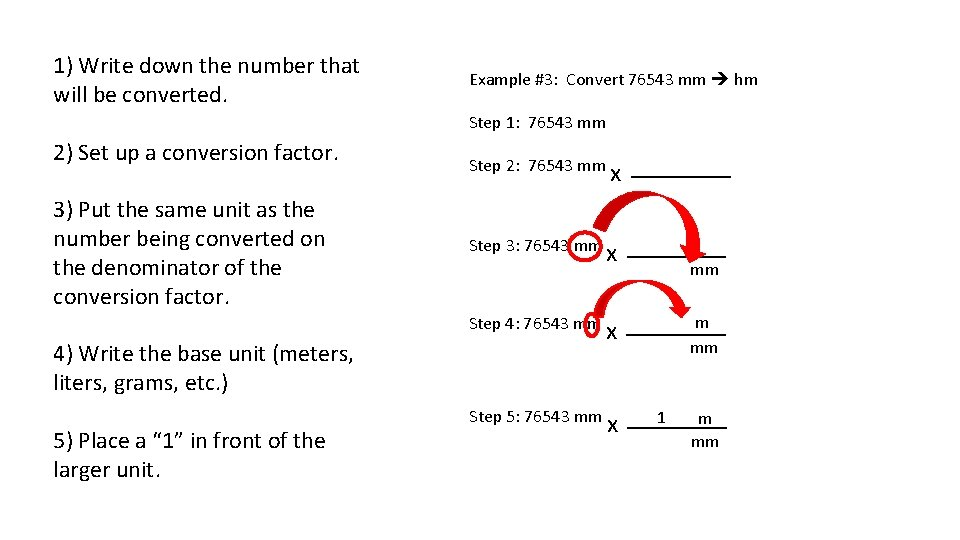1) Write down the number that will be converted. Example #3: Convert 76543 mm hm Step 1: 76543 mm 2) Set up a conversion factor. 3) Put the same unit as the number being converted on the denominator of the conversion factor. Step 2: 76543 mm x _____ Step 3: 76543 mm x _____ x m _____ x _____ 1 m Step 4: 76543 mm 4) Write the base unit (meters, liters, grams, etc. ) 5) Place a “ 1” in front of the larger unit. Step 5: 76543 mm mm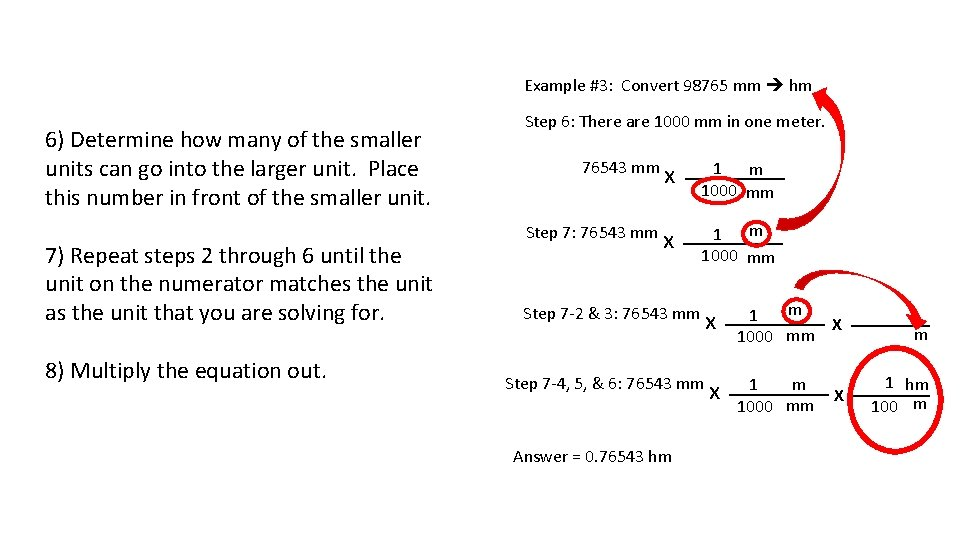Example #3: Convert 98765 mm hm 6) Determine how many of the smaller units can go into the larger unit. Place this number in front of the smaller unit. 7) Repeat steps 2 through 6 until the unit on the numerator matches the unit as the unit that you are solving for. 8) Multiply the equation out. Step 6: There are 1000 mm in one meter. 76543 mm Step 7: 76543 mm x _____ 1 m 1000 mm m _____ 1 x 1000 mm Step 7 -2 & 3: 76543 mm Step 7 -4, 5, & 6: 76543 mm Answer = 0. 76543 hm m _____ 1 x X 1000 mm x m 1 hm _____ m 1 X 1000 mm 100 m